## Absolute Value Inequalities#### Exercise 5.

Find the solutions of the inequality

|2x-5|>x+1.

First we consider the case where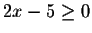, i.e.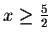. In this case |2x-5|=2x-5, so we can write the inequality as

2x-5>x+1.

Subtracting x on both sides and adding 5, we get x>6.

Now consider the case 2x-5< 0, i.e.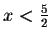. In this case |2x-5|=-(2x-5)=5-2x, so we can write the inequality as

5-2x>x+1.

Adding 2x and subtracting 1 on both sides yields

4>3x,

so we obtain the requirement that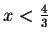.

So a real number x is a solution of the original inequality if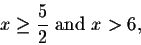or if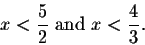Thus the set of solutions is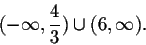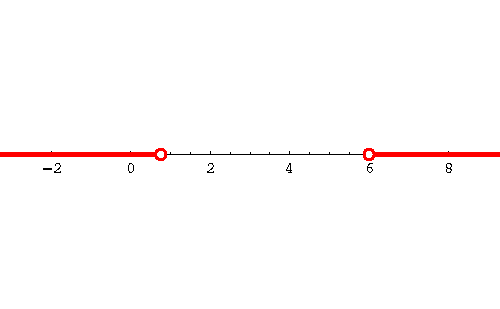[Back] [Exercises] [Next]
[Algebra] [Trigonometry] [Complex Variables]
[Calculus] [Differential Equations] [Matrix Algebra]S.O.S MATHematics home page

Do you need more help? Please post your question on our S.O.S. Mathematics CyberBoard.Helmut Knaust
1998-06-08